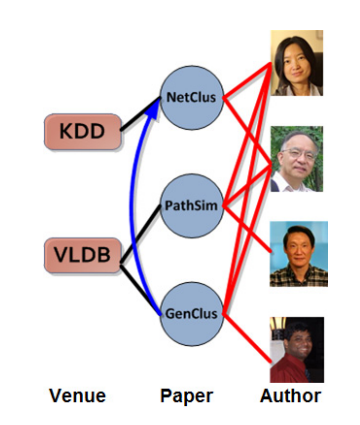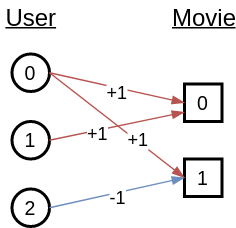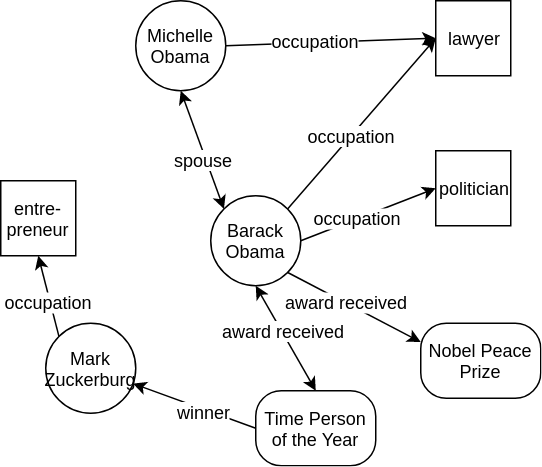# Working with Heterogeneous Graphs¶

Author: Quan Gan, Minjie Wang, Mufei Li, George Karypis, Zheng Zhang

In this tutorial, you learn about:

• Examples of heterogenous graph data and typical applications.
• Creating and manipulating a heterogenous graph in DGL.
• Implementing Relational-GCN, a popular GNN model, for heterogenous graph input.
• Training a model to solve a node classification task.

Heterogeneous graphs, or heterographs for short, are graphs that contain different types of nodes and edges. The different types of nodes and edges tend to have different types of attributes that are designed to capture the characteristics of each node and edge type. Within the context of graph neural networks, depending on their complexity, certain node and edge types might need to be modeled with representations that have a different number of dimensions.

DGL supports graph neural network computations on such heterogeneous graphs, by using the heterograph class and its associated API.

## Examples of heterographs¶

Many graph datasets represent relationships among various types of entities. This section provides an overview for several graph use-cases that show such relationships and can have their data represented as heterographs.

### Citation graph¶

The Association for Computing Machinery publishes an ACM dataset that contains two million papers, their authors, publication venues, and the other papers that were cited. This information can be represented as a heterogeneous graph.

The following diagram shows several entities in the ACM dataset and the relationships among them (taken from Shi et al., 2015).This graph has three types of entities that correspond to papers, authors, and publication venues. It also contains three types of edges that connect the following:

• Authors with papers corresponding to written-by relationships
• Papers with publication venues corresponding to published-in relationships
• Papers with other papers corresponding to cited-by relationships

### Recommender systems¶

The datasets used in recommender systems often contain interactions between users and items. For example, the data could include the ratings that users have provided to movies. Such interactions can be modeled as heterographs.

The nodes in these heterographs will have two types, users and movies. The edges will correspond to the user-movie interactions. Furthermore, if an interaction is marked with a rating, then each rating value could correspond to a different edge type. The following diagram shows an example of user-item interactions as a heterograph.### Knowledge graph¶

Knowledge graphs are inherently heterogenous. For example, in Wikidata, Barack Obama (item Q76) is an instance of a human, which could be viewed as the entity class, whose spouse (item P26) is Michelle Obama (item Q13133) and occupation (item P106) is politician (item Q82955). The relationships are shown in the following. diagram.## Creating a heterograph in DGL¶

You can create a heterograph in DGL using the dgl.heterograph() API. The argument to dgl.heterograph() is a dictionary. The keys are tuples in the form of (srctype, edgetype, dsttype) specifying the relation name and the two entity types it connects. Such tuples are called canonical edge types. The values are data to initialize the graph structures, that is, which nodes the edges actually connect.

For instance, the following code creates the user-item interactions heterograph shown earlier.

# Each value of the dictionary is a pair of source and destination arrays.
# Nodes are integer IDs starting from zero. Nodes IDs of different types have
# separate countings.
import dgl
import numpy as np

ratings = dgl.heterograph(
{('user', '+1', 'movie') : (np.array([0, 0, 1]), np.array([0, 1, 0])),
('user', '-1', 'movie') : (np.array(), np.array())})


DGL supports creating a graph from a variety of data sources. The following code creates the same graph as the above.

Creating from scipy matrix

import scipy.sparse as sp
plus1 = sp.coo_matrix(([1, 1, 1], ([0, 0, 1], [0, 1, 0])), shape=(3, 2))
minus1 = sp.coo_matrix((, (, )), shape=(3, 2))
ratings = dgl.heterograph(
{('user', '+1', 'movie') : plus1,
('user', '-1', 'movie') : minus1})

# Creating from networkx graph
import networkx as nx
plus1 = nx.DiGraph()
plus1.add_edges_from([('u0', 'm0'), ('u0', 'm1'), ('u1', 'm0')])
# To simplify the example, reuse the minus1 object.
# This also means that you could use different sources of graph data
# for different relationships.
ratings = dgl.heterograph(
{('user', '+1', 'movie') : plus1,
('user', '-1', 'movie') : minus1})


## Manipulating heterograph¶

You can create a more realistic heterograph using the ACM dataset. To do this, first download the dataset as follows:

import scipy.io
import urllib.request

data_url = 'https://data.dgl.ai/dataset/ACM.mat'
data_file_path = '/tmp/ACM.mat'

urllib.request.urlretrieve(data_url, data_file_path)
print(list(data.keys()))


Out:

['__header__', '__version__', '__globals__', 'TvsP', 'PvsA', 'PvsV', 'AvsF', 'VvsC', 'PvsL', 'PvsC', 'A', 'C', 'F', 'L', 'P', 'T', 'V', 'PvsT', 'CNormPvsA', 'RNormPvsA', 'CNormPvsC', 'RNormPvsC', 'CNormPvsT', 'RNormPvsT', 'CNormPvsV', 'RNormPvsV', 'CNormVvsC', 'RNormVvsC', 'CNormAvsF', 'RNormAvsF', 'CNormPvsL', 'RNormPvsL', 'stopwords', 'nPvsT', 'nT', 'CNormnPvsT', 'RNormnPvsT', 'nnPvsT', 'nnT', 'CNormnnPvsT', 'RNormnnPvsT', 'PvsP', 'CNormPvsP', 'RNormPvsP']


The dataset stores node information by their types: P for paper, A for author, C for conference, L for subject code, and so on. The relationships are stored as SciPy sparse matrix under key XvsY, where X and Y could be any of the node type code.

The following code prints out some statistics about the paper-author relationships.

print(type(data['PvsA']))
print('#Papers:', data['PvsA'].shape)
print('#Authors:', data['PvsA'].shape)


Out:

<class 'scipy.sparse.csc.csc_matrix'>
#Papers: 12499
#Authors: 17431


Converting this SciPy matrix to a heterograph in DGL is straightforward.

pa_g = dgl.heterograph({('paper', 'written-by', 'author') : data['PvsA']})
# equivalent (shorter) API for creating heterograph with two node types:
pa_g = dgl.bipartite(data['PvsA'], 'paper', 'written-by', 'author')


You can easily print out the type names and other structural information.

print('Node types:', pa_g.ntypes)
print('Edge types:', pa_g.etypes)
print('Canonical edge types:', pa_g.canonical_etypes)

# Nodes and edges are assigned integer IDs starting from zero and each type has its own counting.
# To distinguish the nodes and edges of different types, specify the type name as the argument.
print(pa_g.number_of_nodes('paper'))
# Canonical edge type name can be shortened to only one edge type name if it is
# uniquely distinguishable.
print(pa_g.number_of_edges(('paper', 'written-by', 'author')))
print(pa_g.number_of_edges('written-by'))
print(pa_g.successors(1, etype='written-by'))  # get the authors that write paper #1

# Type name argument could be omitted whenever the behavior is unambiguous.
print(pa_g.number_of_edges())  # Only one edge type, the edge type argument could be omitted


Out:

Node types: ['paper', 'author']
Edge types: ['written-by']
Canonical edge types: [('paper', 'written-by', 'author')]
12499
37055
37055
tensor([3532, 6421, 8516, 8560])
37055


A homogeneous graph is just a special case of a heterograph with only one type of node and edge. In this case, all the APIs are exactly the same as in DGLGraph.

# Paper-citing-paper graph is a homogeneous graph
pp_g = dgl.heterograph({('paper', 'citing', 'paper') : data['PvsP']})
# equivalent (shorter) API for creating homogeneous graph
pp_g = dgl.graph(data['PvsP'], 'paper', 'cite')

# All the ntype and etype arguments could be omitted because the behavior is unambiguous.
print(pp_g.number_of_nodes())
print(pp_g.number_of_edges())
print(pp_g.successors(3))


Out:

12499
30789
tensor([1361, 2624, 8670, 9845])


Create a subset of the ACM graph using the paper-author, paper-paper, and paper-subject relationships. Meanwhile, also add the reverse relationship to prepare for the later sections.

G = dgl.heterograph({
('paper', 'written-by', 'author') : data['PvsA'],
('author', 'writing', 'paper') : data['PvsA'].transpose(),
('paper', 'citing', 'paper') : data['PvsP'],
('paper', 'cited', 'paper') : data['PvsP'].transpose(),
('subject', 'has', 'paper') : data['PvsL'].transpose(),
})

print(G)


Out:

Graph(num_nodes={'author': 17431, 'paper': 12499, 'subject': 73},
num_edges={('paper', 'written-by', 'author'): 37055, ('author', 'writing', 'paper'): 37055, ('paper', 'citing', 'paper'): 30789, ('paper', 'cited', 'paper'): 30789, ('paper', 'is-about', 'subject'): 12499, ('subject', 'has', 'paper'): 12499},
metagraph=[('author', 'paper'), ('paper', 'author'), ('paper', 'paper'), ('paper', 'paper'), ('paper', 'subject'), ('subject', 'paper')])


Metagraph (or network schema) is a useful summary of a heterograph. Serving as a template for a heterograph, it tells how many types of objects exist in the network and where the possible links exist.

DGL provides easy access to the metagraph, which could be visualized using external tools.

# Draw the metagraph using graphviz.
import pygraphviz as pgv
def plot_graph(nxg):
ag = pgv.AGraph(strict=False, directed=True)
for u, v, k in nxg.edges(keys=True):
ag.layout('dot')
ag.draw('graph.png')

plot_graph(G.metagraph)


## Learning tasks associated with heterographs¶

Some of the typical learning tasks that involve heterographs include:

• Node classification and regression to predict the class of each node or estimate a value associated with it.
• Link prediction to predict if there is an edge of a certain type between a pair of nodes, or predict which other nodes a particular node is connected with (and optionally the edge types of such connections).
• Graph classification/regression to assign an entire heterograph into one of the target classes or to estimate a numerical value associated with it.

In this tutorial, we designed a simple example for the first task.

### A semi-supervised node classification example¶

Our goal is to predict the publishing conference of a paper using the ACM academic graph we just created. To further simplify the task, we only focus on papers published in three conferences: KDD, ICML, and VLDB. All the other papers are not labeled, making it a semi-supervised setting.

The following code extracts those papers from the raw dataset and prepares the training, validation, testing split.

import numpy as np
import torch
import torch.nn as nn
import torch.nn.functional as F

pvc = data['PvsC'].tocsr()
# find all papers published in KDD, ICML, VLDB
c_selected = [0, 11, 13]  # KDD, ICML, VLDB
p_selected = pvc[:, c_selected].tocoo()
# generate labels
labels = pvc.indices
labels[labels == 11] = 1
labels[labels == 13] = 2
labels = torch.tensor(labels).long()

# generate train/val/test split
pid = p_selected.row
shuffle = np.random.permutation(pid)
train_idx = torch.tensor(shuffle[0:800]).long()
val_idx = torch.tensor(shuffle[800:900]).long()
test_idx = torch.tensor(shuffle[900:]).long()


### Relational-GCN on heterograph¶

We use Relational-GCN to learn the representation of nodes in the graph. Its message-passing equation is as follows:

$h_i^{(l+1)} = \sigma\left(\sum_{r\in \mathcal{R}} \sum_{j\in\mathcal{N}_r(i)}W_r^{(l)}h_j^{(l)}\right)$

Breaking down the equation, you see that there are two parts in the computation.

1. Message computation and aggregation within each relation $$r$$
2. Reduction that merges the results from multiple relationships

Following this intuition, perform message passing on a heterograph in two steps.

1. Per-edge-type message passing
2. Type wise reduction
import dgl.function as fn

class HeteroRGCNLayer(nn.Module):
def __init__(self, in_size, out_size, etypes):
super(HeteroRGCNLayer, self).__init__()
# W_r for each relation
self.weight = nn.ModuleDict({
name : nn.Linear(in_size, out_size) for name in etypes
})

def forward(self, G, feat_dict):
# The input is a dictionary of node features for each type
funcs = {}
for srctype, etype, dsttype in G.canonical_etypes:
# Compute W_r * h
Wh = self.weight[etype](feat_dict[srctype])
# Save it in graph for message passing
G.nodes[srctype].data['Wh_%s' % etype] = Wh
# Specify per-relation message passing functions: (message_func, reduce_func).
# Note that the results are saved to the same destination feature 'h', which
# hints the type wise reducer for aggregation.
funcs[etype] = (fn.copy_u('Wh_%s' % etype, 'm'), fn.mean('m', 'h'))
# Trigger message passing of multiple types.
# The first argument is the message passing functions for each relation.
# The second one is the type wise reducer, could be "sum", "max",
# "min", "mean", "stack"
G.multi_update_all(funcs, 'sum')
# return the updated node feature dictionary
return {ntype : G.nodes[ntype].data['h'] for ntype in G.ntypes}


Create a simple GNN by stacking two HeteroRGCNLayer. Since the nodes do not have input features, make their embeddings trainable.

class HeteroRGCN(nn.Module):
def __init__(self, G, in_size, hidden_size, out_size):
super(HeteroRGCN, self).__init__()
# Use trainable node embeddings as featureless inputs.
embed_dict = {ntype : nn.Parameter(torch.Tensor(G.number_of_nodes(ntype), in_size))
for ntype in G.ntypes}
for key, embed in embed_dict.items():
nn.init.xavier_uniform_(embed)
self.embed = nn.ParameterDict(embed_dict)
# create layers
self.layer1 = HeteroRGCNLayer(in_size, hidden_size, G.etypes)
self.layer2 = HeteroRGCNLayer(hidden_size, out_size, G.etypes)

def forward(self, G):
h_dict = self.layer1(G, self.embed)
h_dict = {k : F.leaky_relu(h) for k, h in h_dict.items()}
h_dict = self.layer2(G, h_dict)
# get paper logits
return h_dict['paper']


### Train and evaluate¶

Train and evaluate this network.

# Create the model. The output has three logits for three classes.
model = HeteroRGCN(G, 10, 10, 3)

best_val_acc = 0
best_test_acc = 0

for epoch in range(100):
logits = model(G)
# The loss is computed only for labeled nodes.
loss = F.cross_entropy(logits[train_idx], labels[train_idx])

pred = logits.argmax(1)
train_acc = (pred[train_idx] == labels[train_idx]).float().mean()
val_acc = (pred[val_idx] == labels[val_idx]).float().mean()
test_acc = (pred[test_idx] == labels[test_idx]).float().mean()

if best_val_acc < val_acc:
best_val_acc = val_acc
best_test_acc = test_acc

loss.backward()
opt.step()

if epoch % 5 == 0:
print('Loss %.4f, Train Acc %.4f, Val Acc %.4f (Best %.4f), Test Acc %.4f (Best %.4f)' % (
loss.item(),
train_acc.item(),
val_acc.item(),
best_val_acc.item(),
test_acc.item(),
best_test_acc.item(),
))


Out:

Loss 1.0906, Train Acc 0.3150, Val Acc 0.3600 (Best 0.3600), Test Acc 0.2889 (Best 0.2889)
Loss 0.9559, Train Acc 0.5050, Val Acc 0.4600 (Best 0.4600), Test Acc 0.5117 (Best 0.5159)
Loss 0.8022, Train Acc 0.5150, Val Acc 0.4600 (Best 0.4600), Test Acc 0.5142 (Best 0.5159)
Loss 0.5985, Train Acc 0.7900, Val Acc 0.6700 (Best 0.6700), Test Acc 0.7085 (Best 0.7085)
Loss 0.4114, Train Acc 0.8913, Val Acc 0.7300 (Best 0.7300), Test Acc 0.7169 (Best 0.7169)
Loss 0.2712, Train Acc 0.9775, Val Acc 0.7100 (Best 0.7300), Test Acc 0.7504 (Best 0.7169)
Loss 0.1764, Train Acc 0.9962, Val Acc 0.7300 (Best 0.7300), Test Acc 0.7889 (Best 0.7169)
Loss 0.1136, Train Acc 0.9962, Val Acc 0.7300 (Best 0.7400), Test Acc 0.7856 (Best 0.7881)
Loss 0.0737, Train Acc 1.0000, Val Acc 0.7200 (Best 0.7400), Test Acc 0.7864 (Best 0.7881)
Loss 0.0523, Train Acc 1.0000, Val Acc 0.7200 (Best 0.7400), Test Acc 0.7889 (Best 0.7881)
Loss 0.0411, Train Acc 1.0000, Val Acc 0.6900 (Best 0.7400), Test Acc 0.7873 (Best 0.7881)
Loss 0.0346, Train Acc 1.0000, Val Acc 0.6900 (Best 0.7400), Test Acc 0.7923 (Best 0.7881)
Loss 0.0302, Train Acc 1.0000, Val Acc 0.7000 (Best 0.7400), Test Acc 0.7940 (Best 0.7881)
Loss 0.0266, Train Acc 1.0000, Val Acc 0.7000 (Best 0.7400), Test Acc 0.7973 (Best 0.7881)
Loss 0.0235, Train Acc 1.0000, Val Acc 0.7000 (Best 0.7400), Test Acc 0.7948 (Best 0.7881)
Loss 0.0212, Train Acc 1.0000, Val Acc 0.7000 (Best 0.7400), Test Acc 0.7906 (Best 0.7881)
Loss 0.0193, Train Acc 1.0000, Val Acc 0.6900 (Best 0.7400), Test Acc 0.7873 (Best 0.7881)
Loss 0.0177, Train Acc 1.0000, Val Acc 0.6800 (Best 0.7400), Test Acc 0.7881 (Best 0.7881)
Loss 0.0163, Train Acc 1.0000, Val Acc 0.6900 (Best 0.7400), Test Acc 0.7839 (Best 0.7881)
Loss 0.0152, Train Acc 1.0000, Val Acc 0.7000 (Best 0.7400), Test Acc 0.7772 (Best 0.7881)


## What’s next?¶

Total running time of the script: ( 0 minutes 7.454 seconds)

Gallery generated by Sphinx-Gallery